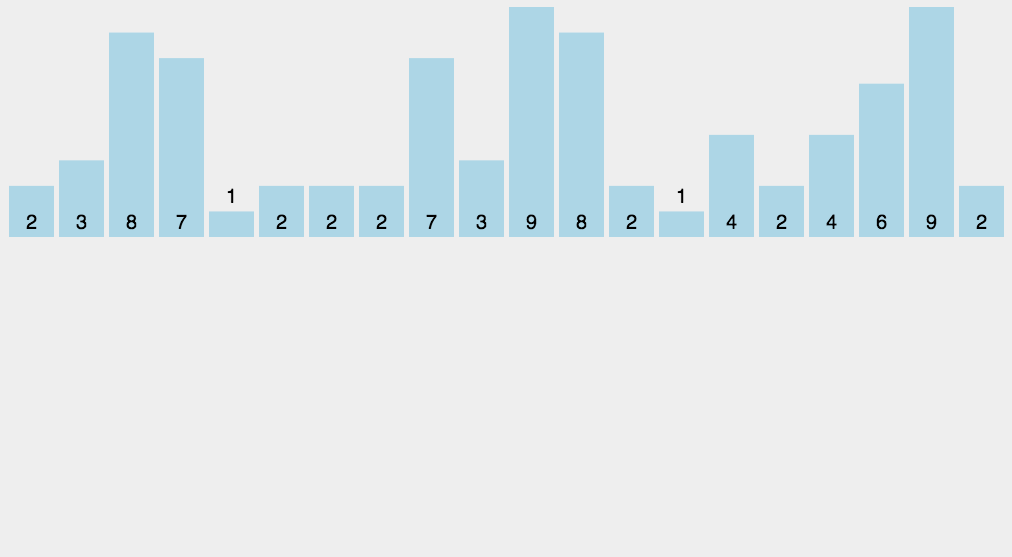# 计数排序（Counting Sort）

## 算法描述

• 找出待排序的数组中最大和最小的元素；
• 统计数组中每个值为i的元素出现的次数，存入数组C的第i项；
• 对所有的计数累加（从C中的第一个元素开始，每一项和前一项相加）；
• 反向填充目标数组：将每个元素i放在新数组的第C(i)项，每放一个元素就将C(i)减去1。

## 动图演示## 代码实现

public static void main(String[] args) {
int[] array = {3, 44, 38, 5, 47, 15, 36, 26, 27, 2, 46, 4, 19, 50, 48};
// 只需要修改成对应的方法名就可以了
countingSort(array);

System.out.println(Arrays.toString(array));
}

/**
* Description: 计数排序
*
* @param array
* @return void
* @author JourWon
* @date 2019/7/11 23:42
*/
public static void countingSort(int[] array) {
if (array == null || array.length <= 1) {
return;
}

int length = array.length;

int max = array;
int min = array;
for (int i = 0; i < length; i++) {
if (max < array[i]) {
max = array[i];
}
if (min > array[i]) {
min = array[i];
}
}
// 最大最小元素之间范围[min, max]的长度
int offset = max - min + 1;
// 1. 计算频率，在需要的数组长度上额外加1
int[] count = new int[offset + 1];
for (int i = 0; i < length; i++) {
// 使用加1后的索引，有重复的该位置就自增
count[array[i] - min + 1]++;
}
// 2. 频率 -> 元素的开始索引
for (int i = 0; i < offset; i++) {
count[i + 1] += count[i];
}

// 3. 元素按照开始索引分类，用到一个和待排数组一样大临时数组存放数据
int[] aux = new int[length];
for (int i = 0; i < length; i++) {
// 填充一个数据后，自增，以便相同的数据可以填到下一个空位
aux[count[array[i] - min]++] = array[i];
}
// 4. 数据回写
for (int i = 0; i < length; i++) {
array[i] = aux[i];
}
}


## 算法分析

11-16566710-095854
05-306040
03-121万+
07-168181
09-16220
02-0361
01-208073